# Probability of Backtest Overfitting

Following the method of Bailey et al., computes for a collection of candidate models the probability of backtest overfitting, the performance degradation and probability of loss, and the stochastic dominance.Implements in R some of the ideas found in the Bailey et al. paper identified below. In particular we use combinatorially symmetric cross validation (CSCV) to implement strategy performance tests evaluated by the Omega ratio. We compute the probability of backtest overfit, performance degradation, probability of loss, and stochastic dominance. We plot visual representations of these using the `lattice` package.

The reference authors used the Sharpe ratio as the performance measure. Other measures are suitable according to the assumptions laid out in the paper.

Example plots attached below. The first four illustrate a test with low overfitting (T-distribution, N=100, T=1600, S=8). The second four illustrate a test from the reference paper with high overfitting (normal distribution, N=100, T=1000, S=8). The third batch illustrate some study selection performance plots for both cases.

Example test case, low overfitting: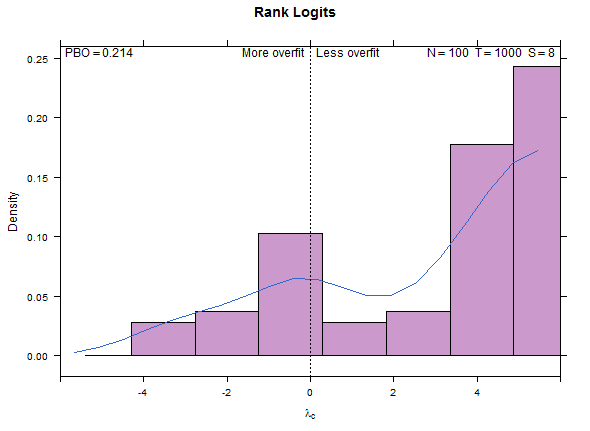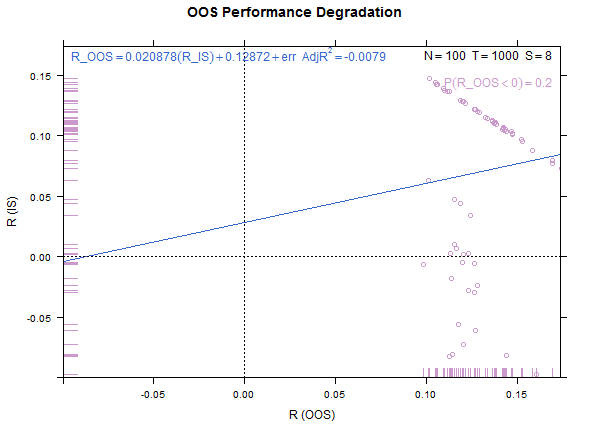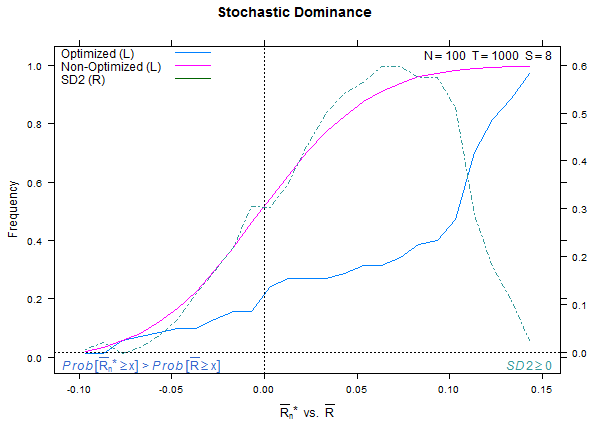Reference test case 1, high overfitting: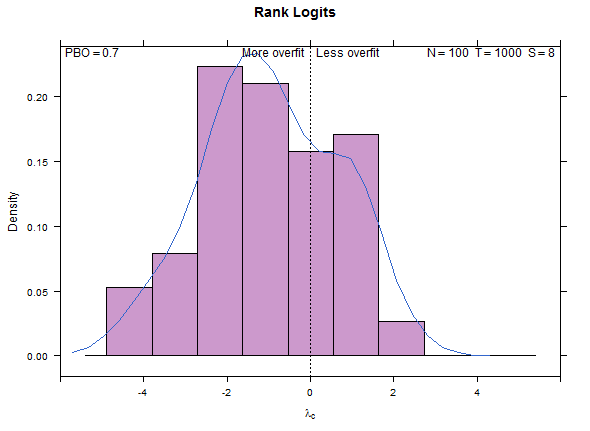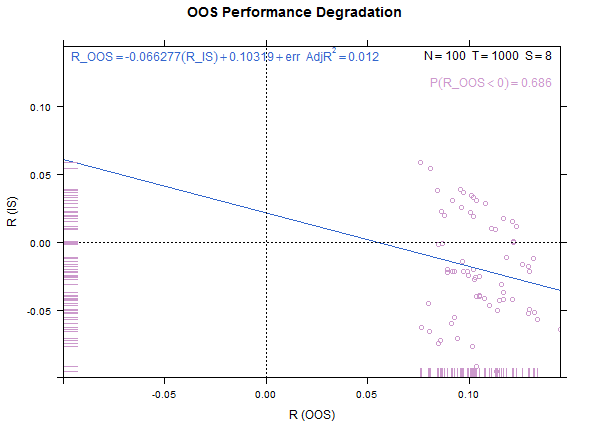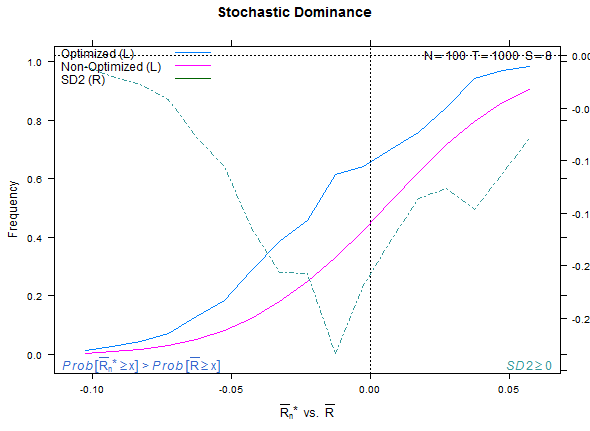Example study selection performance for the low and high cases: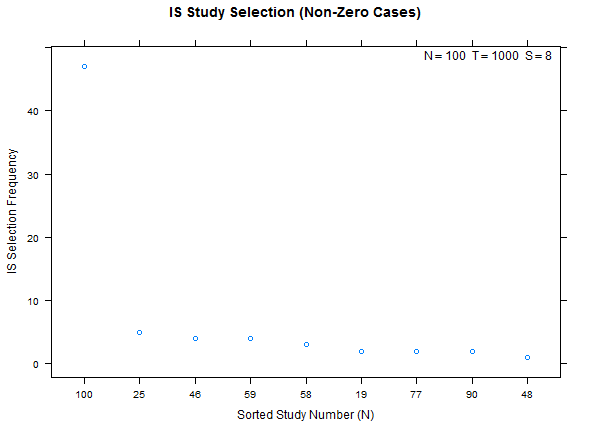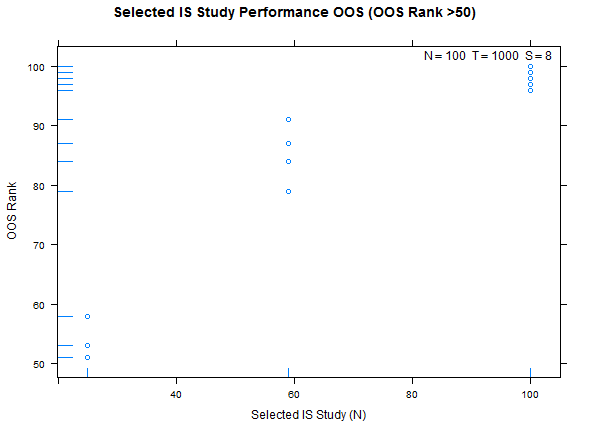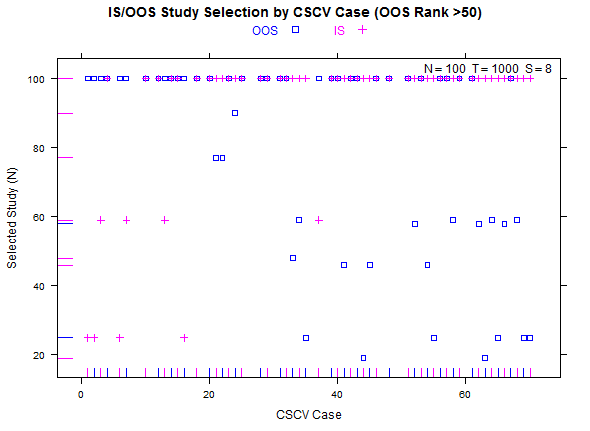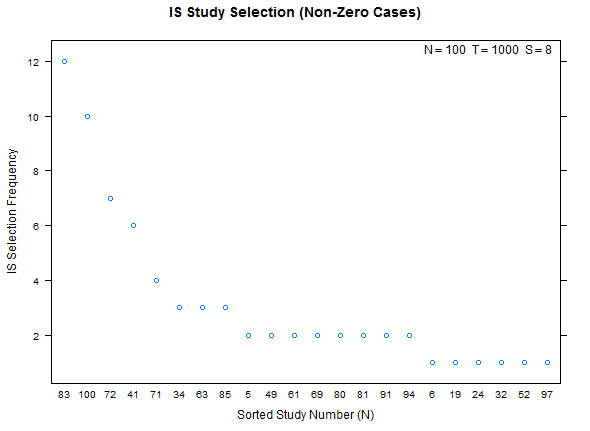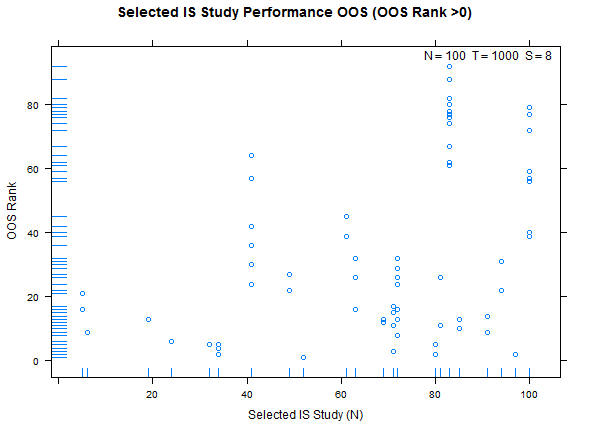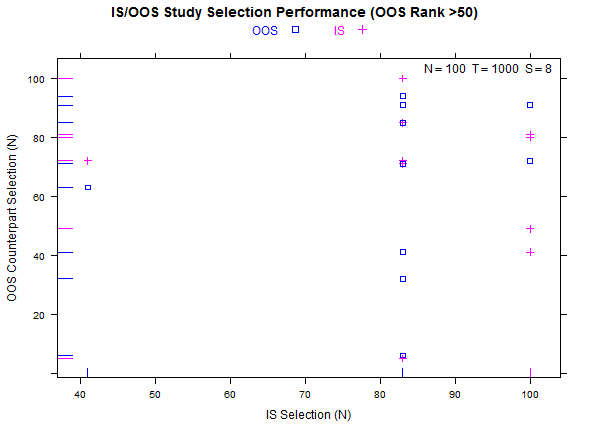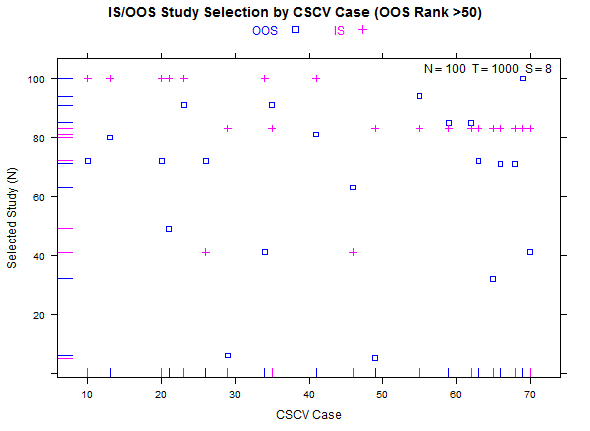More examples with a larger number of combinations on the same high- and low-overfitting test cases. There are 12,780 CSCV combinations with the these tests (normal distribution, N=200, T=2000, S=16, Omega ratio performance).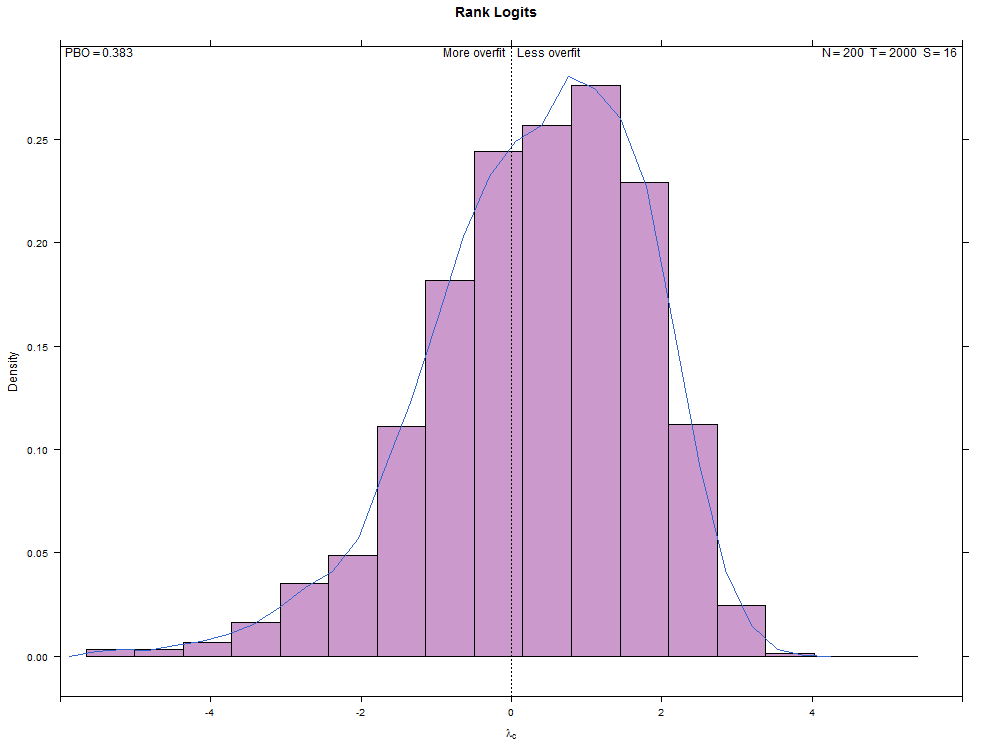## Packages

• `utils` for the combinations
• `lattice` for plots
• `latticeExtra` over plot overlays only for the SD2 measure
• `grid` for plot labeling
• `foreach` for parallel computation of the backtest folds

## Reference

Bailey, David H. and Borwein, Jonathan M. and Lopez de Prado, Marcos and Zhu, Qiji Jim, The Probability of Back-Test Overfitting (September 1, 2013). Available at SSRN: http://ssrn.com/abstract=2326253 or http://dx.doi.org/10.2139/ssrn.2326253

# Reference manual

install.packages("pbo")

1.3.4 by Matt Barry, 7 years ago

https://github.com/mrbcuda/pbo

Report a bug at https://github.com/mrbcuda/pbo/issues

Browse source code at https://github.com/cran/pbo

Authors: Matt Barry <[email protected]>

Documentation:   PDF Manual